## Eigenfunctions and Vector Space

Wavefunctions are analogous to vectors in 3D space. The unit vectors of our vector space are eigenstates.

In normal 3D space, we represent a vector by its components.The unit vectorsare orthonormal,whereis the usual Kroneker delta, equal to 1 if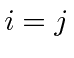and otherwise equal to zero.

Eigenfunctions - the unit vectors of our space - are orthonormal.We represent our wavefunctions - the vectors in our space - as linear combinations of the eigenstates (unit vectors).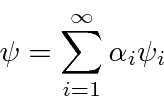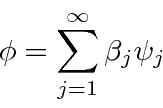In normal 3D space, we can compute the dot product between two vectors using the components.In our vector space, we define the dot product to beWe also can compute the dot product from the components of the vectors. Our vector space is a little bit different because of the complex conjugate involved in the definition of our dot product.

From a more mathematical point of view, the square integrable functions form a (vector) Hilbert Space. The scalar product is defined as above.The properties of the scalar product are easy to derive from the integral.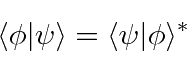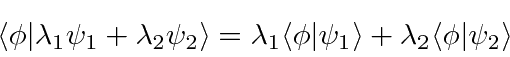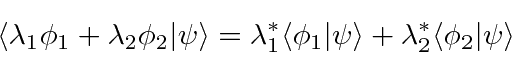is real and greater than 0. It equals zero iff. We may also derive the Schwartz inequality.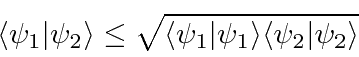Linear operators take vectors in the space into other vectors.Jim Branson 2013-04-22